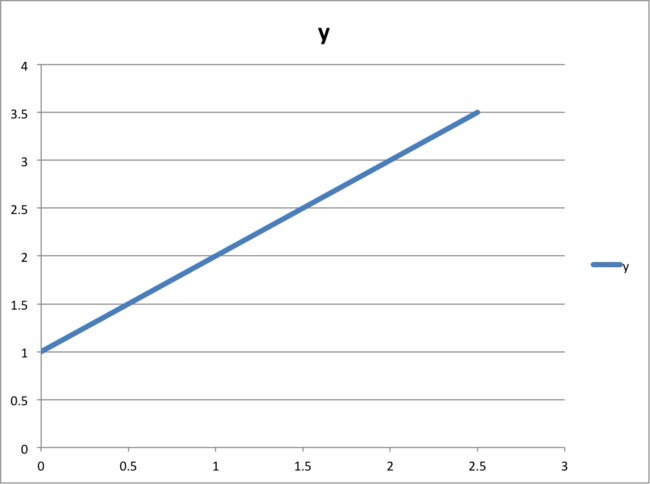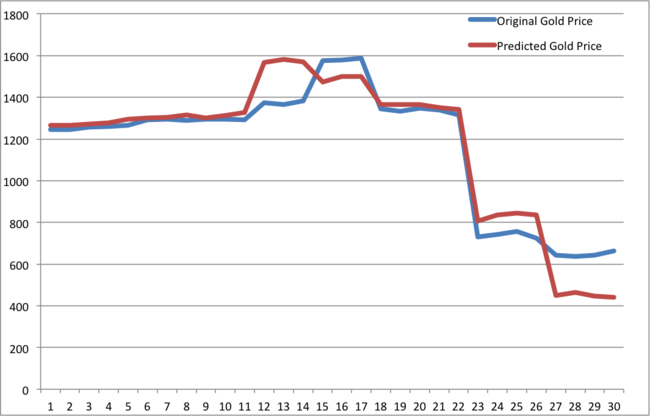Predicting Gold Price - Experiment with machine learning algorithms

Machine Learning often applied to predict unknown values based on historical data available. Using simple ML models prediction become simple if right influencing features are chosen.

For example Gold prices are influenced by following factors,

1. Gold Production
2. Industrial / Consumer Demands (India and China play major role in consumer Jewellery demands)
3. Economic/political situation in the world (Gold is often looked at stable investment options to offset outcome of other volatile financial investment options like stocks).

Features:
So historical values of following parameters are chosen as features to predict gold price

1. World Gold Production in tonnes.
2. Value of Dollar against major currencies.
3. Crude Oil Rate
4. DOW, Nasdaq, SP 500 historical data.

Model:
There are many Machine Learning models (algorithms) are available to choose from, but I used simple Linear Regression Model.

Just to explain how the model works, assume gold price (y), is influenced by factor X, and plotting historical data of X and y in 2D plot may look like below.In order to predict value of gold price y, for values of X, which was not known earlier, drawing a straight line up from the value x axis till the middle line, and horizontal line corresponding to value of y axis will give the expected value. This model fit with algorithm of

y = 1 + x.

This become complicated if the equation become polynomial and many features (each feature is a dimension) are needed to predict (e-g y is function X, Z, W etc). (Even visualising a multidimensional model is beyond human comprehension, we can visualise values of y for given x in 2D space, or at the max in 3D space, but not beyond that).

But the Linear regression models are here to solve these kind of problems, basically linear regression models fit many training (historical data) samples with given features to a mathematical equation which is optimised to predict value y.

Before going next level of details, the final output of Gold Prices vs original price looked like below.Even with simple basic very well known features (historical data available), simple linear regression model really predicted Gold prices very close to the original price. By the way these models are not optimised for accuracy yet.

The training data is here.

Any other better ML models any one like to try with these data, feel free to leave your comment, on your accuracy of output. I used few training samples as my test data to validate my model accuracy.